# 6.1 Graphical Transformations

The graphicx package also provides commands to rotate, resize, reflect and scale text. They are as follows:

`\rotatebox`[<option list>]{<angle>}{<text>}

Rotates <text> by <angle> (degrees anti-clockwise by default). The optional argument <option list> is a comma-separated list of any of the following options:

• units=<number>

The number of units in one full anti-clockwise rotation. So units=-360 means that <angle> specifies degrees clockwise whereas units=6.283185 means that <angle> is in radians.

• origin=<label>

The point of rotation. The value <label> may contain one from either or both of the two lists: lrc (left, right, centre) and tbB (top, bottom, baseline). Alternatively the origin may be specified using the following two keys:

• x=<dimen>

• y=<dimen>

Example:

base line
`\rotatebox`{45}{Some text}
`\rotatebox`[units=-360]{45}{Some text}
`\rotatebox`[units=-360,origin=rB]{45}{Some text}
`\rotatebox`[x=3em,y=3em]{45}{Some text}
base line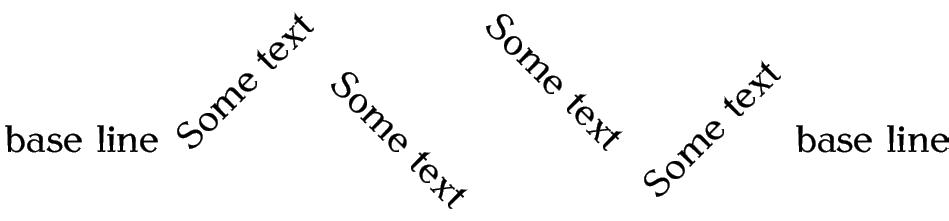`\scalebox`{<h scale>}[<v scale>]{<text>}

Scales <text> by <h scale> in both directions if <v scale> omitted, otherwise scales <text> by <h scale> horizontally and <y scale> vertically.

Example:

`\scalebox`{0.8}{Some text}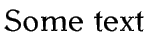Compare with:

`\scalebox`{0.8}[1.2]{Some text}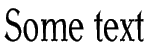`\reflectbox`{<text>}

Reflects <text> (equivalent to `\scalebox`{-1}{<text>}).

Example:

`\reflectbox`{Some text}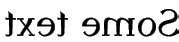`\resizebox`{<h length>}{<v length>}{<text>}

Scales <text> so that it is <h length> wide and <v length> high. To preserve the aspect ratio, use ! instead of one of the dimensions.

Example: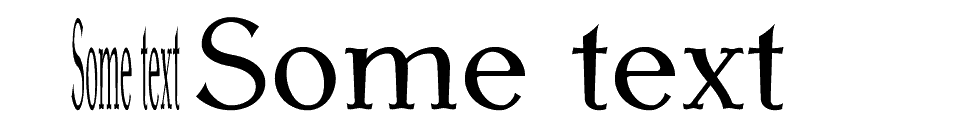This book is also available as A4 PDF or 12.8cm x 9.6cm PDF or paperback (ISBN 978-1-909440-00-5).

© 2012 Dickimaw Books. "Dickimaw", "Dickimaw Books" and the Dickimaw parrot logo are trademarks. The Dickimaw parrot was painted by Magdalene Pritchett.